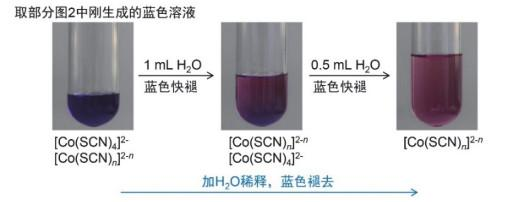## Reunderstanding of the "Identification of Co2+" Experiment: One of the Best Examples of Critical Thinking Education

Lü Yinyun, Ruan Chanzi, Zhang Chunyan, Pan Rui, Xu Zhenling, Ren Yanping,

 基金资助: 2017年福建省本科高校教育教学改革研究项目.  FBJG201702952017年度厦门大学教学改革研究项目.  JG20170204国家基础科学人才培养基金项目.  J1310024Abstract

Taking the experiment of the "identification of Co2+" as an example, this article elaborates the flexible application of "flipped classroom" model in the chemical laboratory teaching. Specifically, students do the experiment first and have questions during the experimental process, then the teacher discusses and demonstrates with students base on their questions, leading students to reunderstand the classic experiment of the "identification of Co2+". Thus this article reveals about how to educate students to think and criticize in the teaching process.

Keywords： Chemical laboratory teaching ; Identification of Co2+ ; Reunderstanding ; Flipped Classroom ; Criticizing

Lü Yinyun. Reunderstanding of the "Identification of Co2+" Experiment: One of the Best Examples of Critical Thinking Education. University Chemistry[J], 2020, 35(9): 89-95 doi:10.3866/PKU.DXHX201909023

## 1 对经典的“ Co2+鉴定”实验的认识

### 图1## 2 由经典的“ Co2+鉴定”实验引发的思考

1) NH4SCN溶液是无色的，在2 mL 0.1 mol∙L−1 CoCl2溶液中加入1 mL 10% NH4SCN溶液，图1中所得体系溶液颜色为什么会变成紫红色？这是我们亲眼看到的实验事实。如果没有新的物种形成的话，2 mL 0.1 mol∙L−1 CoCl2溶液只有被稀释而颜色变浅，而实验事实是溶液颜色变深；在紫红色溶液中加入戊醇有蓝色[Co(SCN)4]2−形成，说明这紫红色物质可能是低级配离子[Co(SCN)n]2−n (n = 1–3)，但目前我们还无法通过实验来证实。学生在具体做实验时，直接加戊醇，忽略了对加戊醇前这步产物的认识与分析。

$\underset{粉红色}{\mathop{{{\left[ \text{Co}{{\left( {{\text{H}}_{\text{2}}}\text{O} \right)}_{6}} \right]}^{2+}}}}\, +\text{SC}{{\text{N}}^{-}}\to \underset{紫红色\left( n=1-3 \right)}{\mathop{{{\left[ \text{Co}{{\left( \text{SCN} \right)}_{n}} \right]}^{2-n}}}}\, \xrightarrow{戊醇}\underset{蓝色}{\mathop{{{\left[ \text{Co}{{\left( \text{SCN} \right)}_{4}} \right]}^{2-}}}}\,$

2)在水溶液中没有观察到蓝色[Co(SCN)4]2−存在，是在给定条件下(温度、浓度一定)根本没有形成蓝色的[Co(SCN)4]2−，而只形成低级配离子[Co(SCN)n]2−n (n = 1–3)，还是像教科书上所描述的那样“形成的[Co(SCN)4]2−在水溶液中不稳定(K = 103)，易离解”而形成低级配离子[Co(SCN)n]2−n (n = 1–3)？改变反应条件，能否在水溶液中形成肉眼可见的蓝色[Co(SCN)4]2−？在水溶液中蓝色的[Co(SCN)4]2−能否稳定存在？

3)加戊醇或丙酮等有机溶剂鉴定Co2+的方法灵敏，且具有唯一性，但有污染、麻烦，能否找到不用有机溶剂，简单、环保而又能有效地来鉴定Co2+的方法？

### 图2### 图3### 图4$\underset{粉红色}{\mathop{{{\left[ \text{Co}{{\left( {{\text{H}}_{\text{2}}}\text{O} \right)}_{6}} \right]}^{2+}}}}\,+\text{SC}{{\text{N}}^{-}}\rightleftarrows \underset{紫红色\left( n=1-3 \right)}{\mathop{{{\left[ \text{Co}{{\left( \text{SCN} \right)}_{n}} \right]}^{2-n}}}}\,\underset{\rm H_2 O}{\overset{戊醇或\text{SC}{{\text{N}}^{-}}}{\rightleftarrows}}\underset{蓝色}{\mathop{{{\left[ \text{Co}{{\left( \text{SCN} \right)}_{4}} \right]}^{2-}}}}\,$

### 3.2 加热

$\underset{粉红色}{\mathop{{{\left[ \text{Co}{{\left( {{\text{H}}_{\text{2}}}\text{O} \right)}_{6}} \right]}^{2+}}}}\,+\text{SC}{{\text{N}}^{-}}\rightleftarrows \underset{紫红色\left( n=1-3 \right)}{\mathop{{{\left[ \text{Co}{{\left( \text{SCN} \right)}_{n}} \right]}^{2-n}}}}\,\underset{冷却}{\overset{\Delta}{\rightleftarrows}}\underset{蓝色}{\mathop{{{\left[ \text{Co}{{\left( \text{SCN} \right)}_{4}} \right]}^{2-}}}}\,$

### 图5### 图6### 图7### 图8## 参考文献 原文顺序 文献年度倒序 文中引用次数倒序 被引期刊影响因子

/

 〈〉